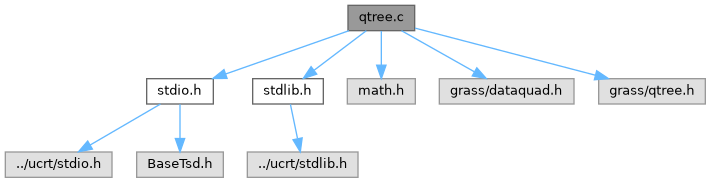GRASS GIS 8 Programmer's Manual  8.2.2dev(2023)-5c00b4e944
qtree.c File Reference
`#include <stdio.h>`
`#include <stdlib.h>`
`#include <math.h>`
`#include <grass/dataquad.h>`
`#include <grass/qtree.h>`
Include dependency graph for qtree.c:Go to the source code of this file.

## Functions

struct tree_infoMT_tree_info_new (struct multtree *root, struct multfunc *functions, double dmin, int kmax)

struct multtreeMT_tree_new (struct quaddata *data, struct multtree **leafs, struct multtree *parent, int multant)

int MT_insert (struct triple *point, struct tree_info *info, struct multtree *tree, int n_leafs)

int MT_divide (struct tree_info *info, struct multtree *tree, int n_leafs)

int MT_region_data (struct tree_info *info, struct multtree *tree, struct quaddata *data, int MAX, int n_leafs)

## Detailed Description

Definition in file qtree.c.

## ◆ MT_divide()

 int MT_divide ( struct tree_info * info, struct multtree * tree, int n_leafs )

Divide a tree

Divides the tree by calling one of tree's functions (divide_data()) and returns the result of divide_data()

Definition at line 158 of file qtree.c.

Referenced by MT_insert().

## ◆ MT_functions_new()

Initializes multfunc structure with given arguments

Definition at line 33 of file qtree.c.

## ◆ MT_insert()

 int MT_insert ( struct triple * point, struct tree_info * info, struct multtree * tree, int n_leafs )

First checks for dividing cond. (if n_points>=KMAX) and tree is a leaf by calling one of tree's functions (`division_check()`). If tree is not a leaf (is a node) uses function compare to determine into which "son" we need to insert the point and calls MT_insert() with this son as a n argument.

If TREE is a leaf but we don't need to divide it (n_points<KMAX) then calls function `add_data(point, ...)` to add point to the data of tree and returns the result of `add_data()` (which returns 1 if the point is inserted and 0 if its ignored (when its too dense)).

If `division_check()` returns true, calls MT_divide() and then calls MT_insert() to insert the point into divided tree and returns the result of MT_divide().

Definition at line 105 of file qtree.c.

## ◆ MT_region_data()

 int MT_region_data ( struct tree_info * info, struct multtree * tree, struct quaddata * data, int MAX, int n_leafs )

Get points inside a region from a tree

Gets points inside the region defined by DATA from TREE and adds them to DATA. If the number of eligible point is more than MAX returns MAX+1 otherwise returns number of points added to DATA.

Uses tree's functions intersect() to find leafs that intersect given region and get_points() to get points from such leafs.

Parameters
 MAX max number of points we can add (KMAX2)

Definition at line 194 of file qtree.c.

## ◆ MT_tree_info_new()

 struct tree_info* MT_tree_info_new ( struct multtree * root, struct multfunc * functions, double dmin, int kmax )

Initializes tree_info using given arguments

Definition at line 57 of file qtree.c.

References tree_info::dmin, tree_info::functions, tree_info::kmax, malloc(), NULL, and tree_info::root.

## ◆ MT_tree_new()

 struct multtree* MT_tree_new ( struct quaddata * data, struct multtree ** leafs, struct multtree * parent, int multant )

Initializes multtree using given arguments

Definition at line 73 of file qtree.c.

Referenced by MT_divide().